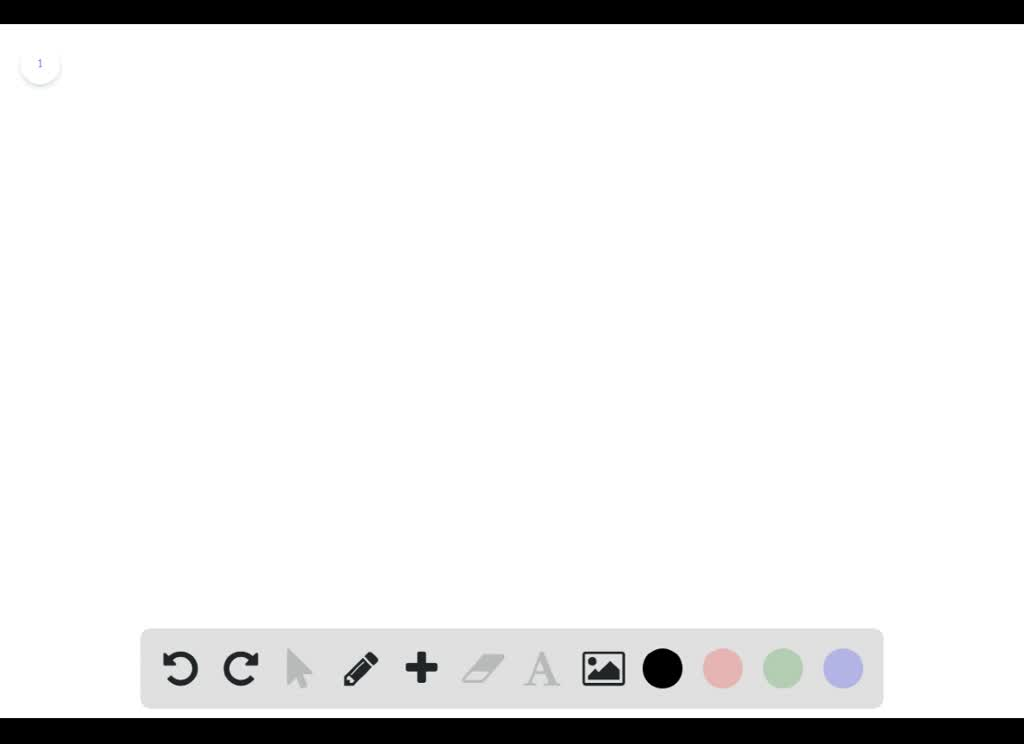5

# 1. Calculate the volume (in L) of O2 at a pressure of744 mm Hg and 32.0 oC that will be consumed by combusting 10.0 gof C15H21N.2.The CO2 produced from the combust...

## Question

###### 1. Calculate the volume (in L) of O2 at a pressure of744 mm Hg and 32.0 oC that will be consumed by combusting 10.0 gof C15H21N.2.The CO2 produced from the combustion of C15H21N iscollected over water at a total pressure of 755 mm Hg, 28.0 oC anda volume of 165 mL. Calculate the partial pressure of CO2 and thegrams of C15H21N consumed

1. Calculate the volume (in L) of O2 at a pressure of 744 mm Hg and 32.0 oC that will be consumed by combusting 10.0 g of C15H21N. 2.The CO2 produced from the combustion of C15H21N is collected over water at a total pressure of 755 mm Hg, 28.0 oC and a volume of 165 mL. Calculate the partial pressure of CO2 and the grams of C15H21N consumed